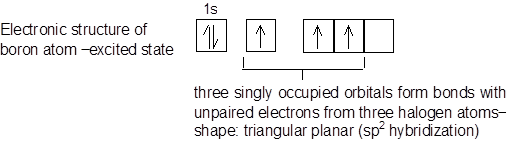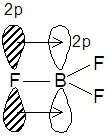# What is the boron trifluoride formula?

## Boron Trifluoride Formula and its structure

To know the Boron Trifluoride Formula we have to understand the shape of the BF3 molecule is a planar triangle with bond angles of 120°. This is predicted by VSEPR theory as the most stable shape for three outer electron pairs around B. The valence bond theory also predicts a planar triangle with hybridisation of one s and two p orbitals used for bonding. However, the B atom only has six electrons in its outer shell and this is termed electron deficient.In Boron Trifluoride Formula the bond lengths in BF3 are 1.30 Å each and are significantly shorter than the sum of the covalent radii  (B = 0.80 Å, F = 0.72 Å). The bond energy is very high: 646 kJ mol−1, which is higher than for any single bond. The shortness and strength of the bonds is interpreted in terms of a pπ−pπ interaction, that is the bonds possess some double bond character. The empty 2pz atomic orbital on B which is not involved in hybridisation is perpendicular to the triangle containing the sp2 hybrid orbitals. This pz orbital may accept an electron pair from a full pz orbital on any one of the three fluorine atoms. Thus, a dative π bond is formed and the B atom attains an octet of electrons. If one localized double bond existed, then there would be one short bond and two longer ones. However, all measurements show that the three bond lengths are identical. The old valence bond explanation of this was resonance between three structures with the double bond in different positions. The modern explanation is that the double bond is delocalised. The pz atomic orbitals from B and the three F atoms form a four−centre π molecular orbital covering all four atoms which contains two bonding electrons. Check out Chemistry Formulas and NCERT Solutions for class 11 Chemistry prepared by Physics Wallah.

### Boron Trifluoride Structure### The acidic strength of the trihalides is in order

BBr3>BCl3>  BF3

The order is the reverse of what would be normally expected on the basis of electronegativity of halogen and also on the basis of steric grounds.

This is explained on the basis of B−X π−bonding. The tendency to form the pπ−pπ double bond is maximum in BF3 and decreases very rapidly as we move to BCl3 and BBr3.